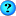View Single Post
#1
 ptjt255Member Join Date: Oct 2008 Posts: 24Semi-circle array containing target and distractor objects

Hi,

I wonder if someone can help me, I am trying to set up a semi-circle array of positions on the left hand side of the screen, depending on how many distractors are selected (e.g. either 2, 4, 6 or 8) each position should contain one target object and the rest distractors, these should be randomised. I then want the target position to be a hotspot. But at this stage I'm having trouble just getting an array of positions where I want them with the correct objects in them.

Any help would be hugely appreciated.

Thank you.

Code:
```import viz
import viztask
import random
viz.go()

Z_dist = -5
#viz.move(0,0,Z_dist)
viz.MainView.move(0.5,0,-10)

# Add distractor and target objects
ballsize = [2.0,2.0,2.0,1.0,1.0,1.0]

# Distractor ball
distractor = viz.add('soccerball.ive')
distractor.setScale(ballsize,ballsize,2)
distractor.addAction(vizact.spin(0,1,0,100))

# Target Ball
target = viz.add('ball.wrl')
target.setScale(ballsize,ballsize,1)
target.addAction(vizact.spin(0,1,0,100))

# Set up zones for the objects to appear on the screen
choice = ['2', '4', '6', '8']
select  = viz.choose('How many distractors?', choice)

#make a list with the objects in it
objects = [distractor,target]

# keep 2 positions in an array
pos = [[-1.0,0.0,Z_dist],[-1.0,0.5,Z_dist],[-2.0,1.0,Z_dist],[-1.0,1.5,Z_dist],[-1.0,2.0,Z_dist],[-1.0,2.5,Z_dist],[-1.0,3.0,Z_dist],[-1.0,3.5,Z_dist]]

rand_positions = []

def sceneOn(num):
global rand_positions
positions = [0,1,2,3,4,5,6,7]
if select == 0:

#set objects to 2 different random positions
rand_positions = random.sample(positions,2)

objects.setPosition(pos[rand_positions])
objects.setPosition(pos[rand_positions])

if select == 1:

#set objects to 4 different random positions
rand_positions = random.sample(positions,4)

objects.setPosition(pos[rand_positions])
objects.setPosition(pos[rand_positions])
objects.setPosition(pos[rand_positions])
objects.setPosition(pos[rand_positions])

if select == 2:

#set objects to 4 different random positions
rand_positions = random.sample(positions,6)

objects.setPosition(pos[rand_positions])
objects.setPosition(pos[rand_positions])
objects.setPosition(pos[rand_positions])
objects.setPosition(pos[rand_positions])
objects.setPosition(pos[rand_positions])
objects.setPosition(pos[rand_positions])

if select == 3:
rand_positions = random.sample(positions,8)

objects.setPosition(pos[rand_positions])
objects.setPosition(pos[rand_positions])
objects.setPosition(pos[rand_positions])
objects.setPosition(pos[rand_positions])
objects.setPosition(pos[rand_positions])
objects.setPosition(pos[rand_positions])
objects.setPosition(pos[rand_positions])
objects.setPosition(pos[rand_positions])

sceneOn(1)```